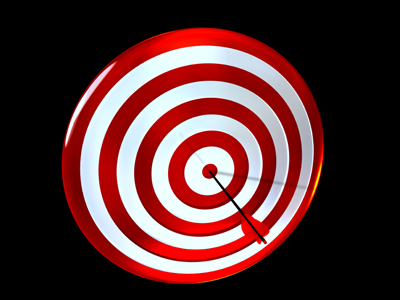The name given to the distance from the centre of a polygon or circle to one of the vertices is radius.

# Shapes (Year 4)

In KS2 Maths, you'll explore cool shapes! Year Four is super exciting because you learn about tricky shapes like heptagons and octagons. Triangles get fancy too, with equilateral ones! We'll chat about 2D shapes (like rectangles) and 3D shapes (like cubes). They call 2D shapes "polygons" and 3D shapes "polyhedrons" – big words, right?

Rectangles and squares are flat polygons, while cuboids and cubes are blocky polyhedrons. There are more words like angles and radius, but don't worry, we'll figure them out together. Ready for a fun quiz on shapes? Let's play!

1.
What is the name of the triangle with all sides and all angles equal?
Right angled triangle
Isosceles triangle
Equilateral triangle
Pyramid
An equilateral triangle has angles of 60o at each of its corners
2.
What is the name given to a shape that has four faces all of which are equilateral triangles?
Tetrahedron
Polyhedron
Prism
Pyramid
Tetrahedrons are similar to pyramids, but pyramids have 5 faces, one of which is a square
3.
What is the name of all flat shapes with three or more straight sides?
Triangle
Polyhedron
Polygon
Heptagon
So triangles, squares, rectangles and pentagons are all polygons
4.
What is the name given to the distance from the centre of a polygon or circle to one of the vertices?
Circumference
Diameter
Tangent
The diameter is twice the radius
5.
How many sides does an octagon have?
5
6
7
8
To help remember this, think of an octopus with 8 legs
6.
Which of these describes an isosceles triangle?
Triangle with 3 equal sides
Triangle with 2 equal sides and 2 equal angles
Triangle with 3 equal sides and 2 equal angles
Triangle with 2 equal sides and 3 equal angles
An isosceles triangle has 2 equal angles which are opposite to 2 sides of equal length
7.
Which of these is a regular polygon?
Rectangle
Right angled triangle
Square
Circle
A regular polygon has all sides equal
8.
What is a polyhedron?
A 3D shape where all faces are square
A 3D shape where all faces are flat polygons
A 2D shape where all angles are different
A 2D shape with twelve sides
Cubes and cuboids are both polyhedrons
9.
What is a seven sided polygon called?
Heptagon
Hexagon
Pentagon
Tetrahedron
A heptagon has 7 sides and a heptagram is a star with 7 points
10.
How many degrees in a right angle?
60o
90o
180o
360o
There are 360o in a full turn so a quarter turn will be 90o
You can find more about this topic by visiting BBC Bitesize - Recognise and describe 3D shapes

Author:  Amanda Swift